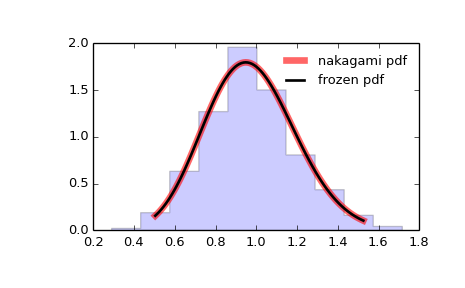# scipy.stats.nakagami¶

scipy.stats.nakagami = <scipy.stats._continuous_distns.nakagami_gen object at 0x4508308c>[source]

A Nakagami continuous random variable.

As an instance of the rv_continuous class, nakagami object inherits from it a collection of generic methods (see below for the full list), and completes them with details specific for this particular distribution.

Notes

The probability density function for nakagami is:

nakagami.pdf(x, nu) = 2 * nu**nu / gamma(nu) *
x**(2*nu-1) * exp(-nu*x**2)


for x > 0, nu > 0.

nakagami takes nu as a shape parameter.

The probability density above is defined in the “standardized” form. To shift and/or scale the distribution use the loc and scale parameters. Specifically, nakagami.pdf(x, nu, loc, scale) is identically equivalent to nakagami.pdf(y, nu) / scale with y = (x - loc) / scale.

Examples

>>> from scipy.stats import nakagami
>>> import matplotlib.pyplot as plt
>>> fig, ax = plt.subplots(1, 1)


Calculate a few first moments:

>>> nu = 4.97
>>> mean, var, skew, kurt = nakagami.stats(nu, moments='mvsk')


Display the probability density function (pdf):

>>> x = np.linspace(nakagami.ppf(0.01, nu),
...                 nakagami.ppf(0.99, nu), 100)
>>> ax.plot(x, nakagami.pdf(x, nu),
...        'r-', lw=5, alpha=0.6, label='nakagami pdf')


Alternatively, the distribution object can be called (as a function) to fix the shape, location and scale parameters. This returns a “frozen” RV object holding the given parameters fixed.

Freeze the distribution and display the frozen pdf:

>>> rv = nakagami(nu)
>>> ax.plot(x, rv.pdf(x), 'k-', lw=2, label='frozen pdf')


Check accuracy of cdf and ppf:

>>> vals = nakagami.ppf([0.001, 0.5, 0.999], nu)
>>> np.allclose([0.001, 0.5, 0.999], nakagami.cdf(vals, nu))
True


Generate random numbers:

>>> r = nakagami.rvs(nu, size=1000)


And compare the histogram:

>>> ax.hist(r, normed=True, histtype='stepfilled', alpha=0.2)
>>> ax.legend(loc='best', frameon=False)
>>> plt.show()Methods

 rvs(nu, loc=0, scale=1, size=1, random_state=None) Random variates. pdf(x, nu, loc=0, scale=1) Probability density function. logpdf(x, nu, loc=0, scale=1) Log of the probability density function. cdf(x, nu, loc=0, scale=1) Cumulative density function. logcdf(x, nu, loc=0, scale=1) Log of the cumulative density function. sf(x, nu, loc=0, scale=1) Survival function (1 - cdf — sometimes more accurate). logsf(x, nu, loc=0, scale=1) Log of the survival function. ppf(q, nu, loc=0, scale=1) Percent point function (inverse of cdf — percentiles). isf(q, nu, loc=0, scale=1) Inverse survival function (inverse of sf). moment(n, nu, loc=0, scale=1) Non-central moment of order n stats(nu, loc=0, scale=1, moments='mv') Mean(‘m’), variance(‘v’), skew(‘s’), and/or kurtosis(‘k’). entropy(nu, loc=0, scale=1) (Differential) entropy of the RV. fit(data, nu, loc=0, scale=1) Parameter estimates for generic data. expect(func, nu, loc=0, scale=1, lb=None, ub=None, conditional=False, **kwds) Expected value of a function (of one argument) with respect to the distribution. median(nu, loc=0, scale=1) Median of the distribution. mean(nu, loc=0, scale=1) Mean of the distribution. var(nu, loc=0, scale=1) Variance of the distribution. std(nu, loc=0, scale=1) Standard deviation of the distribution. interval(alpha, nu, loc=0, scale=1) Endpoints of the range that contains alpha percent of the distribution

#### Previous topic

scipy.stats.mielke

scipy.stats.ncx2